# Bathroom 2

A bathroom is 2.4 meters long and 1.8 meters wide. How many square tiles 1 dm on each side are to be used to cover it?

n =  432

### Step-by-step explanation: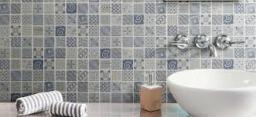Did you find an error or inaccuracy? Feel free to write us. Thank you!#### You need to know the following knowledge to solve this word math problem:

We encourage you to watch this tutorial video on this math problem:

## Related math problems and questions:

• Bathroom 3De Mesa family will soon be occupying their newly renovated house . However the bathroom, measuring 10ft by 16ft, still to be covered with tiles . If the tiles that they desire measures 2/5 ft by 2/5ft, how many tiles will they need to cover the bathroom
• Square tilesThe room has dimensions of 12 meters and 5.6 meters. Determine the number of square tiles and their largest dimension to exactly cover the floor.
• TrevorTrevor wishes to tile the floor of his bathroom. The bathroom is rectangular and is 4.2m long and 3.3m wide. The tiles are 30cm by 30cm. Calculate the cost to tile the floor if each tile costs 72 cents.
• The wall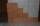The wall in the bathroom has dimensions of 2.8 m and 2.6 m. If we would like to cover this wall to a height of 1.6 m. How many square meters of tiles do we need to buy?
• In the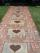In the garden, workers will pave a 1-meter-wide sidewalk with tiles around the block-shaped pool. The dimensions of the bottom of the pool are 8.5 meters and 6 meters. The height of the pool walls is 2 meters. How many m2 of pavement will be laid with til
• TilesThe room has dimensions 12 m and 5.6 m. Determine the number of square tiles and their largest possible size to cover them room's floor.
• The rugJosie has a rug with the area of 18 square feet. She will put the rug on the floor that is covered in 1/3 square foot tiles. How many tiles will the rug cover?
• Tiles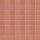How many 46 cm square tiles will cover a floor 22.08 m by 8.74 m?
• The corridor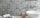The corridor is 12 m long and 3.6 m wide. It must be paved with rectangular tiles measuring 15 cm and 30 cm. Are 1000 pieces of tiles enough to pave the corridor?
• RectangleThe rectangle area is 182 dm2, its base is 14 dm. How long is the other side? Calculate its perimeter.
• Tiles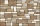The rectangular floor of the 6 x 1.8 m dimensions is to be covered with 50 cm square tiles. How many tiles will be needed?
• Pool tilesThe pool is 25m long, 10m wide, and 160cm deep. How many m2 of tiles will be needed on the walls and the pool? How many tiles are needed when 1 tile has a square shape with a 20cm side? How much does it cost when 1m2 of tiles costs 258 Kc?
• TilesHall has dimensions 325 &time; 170 dm. What is the largest size of square tiles that can be entire hall tiled, and how many we need them?
• The carpet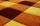How many meters of carpet 90 cm wide need to cover floor room which has a rectangular shape with a lengths 4.8 m and 2.4 m if the number of pieces on the carpet is needed to be lowest?
• Water depthHow many square meters of the inside of the pool wet the water if the pool is 25 meters long and 10 meters wide and the water depth is 1.2 meters everywhere.
• Long and wideWhat is the area of a rectangle that is 1 1/4 meters long and 3/4​ of a meter wide
• Parallelogram - sideCalculate the length of the side of a parallelogram whose area is 175 dm2 and height to that side is 24.2 dm long.